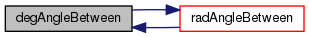The OpenFOAM Foundation
Foam::vectorTools Namespace Reference

Collection of functions for testing relationships between two vectors. More...

## Functions

template<class T >
bool areParallel (const Vector< T > &a, const Vector< T > &b, const T &tolerance=small)
Test if a and b are parallel: a^b = 0. More...

template<class T >
bool areOrthogonal (const Vector< T > &a, const Vector< T > &b, const T &tolerance=small)
Test if a and b are orthogonal: a.b = 0. More...

template<class T >
bool areAcute (const Vector< T > &a, const Vector< T > &b)
Test if angle between a and b is acute: a.b > 0. More...

template<class T >
bool areObtuse (const Vector< T > &a, const Vector< T > &b)
Test if angle between a and b is obtuse: a.b < 0. More...

template<class T >
T cosPhi (const Vector< T > &a, const Vector< T > &b, const T &tolerance=small)
Calculate angle between a and b in radians. More...

template<class T >
T radAngleBetween (const Vector< T > &a, const Vector< T > &b, const T &tolerance=small)
Calculate angle between a and b in radians. More...

template<class T >
T degAngleBetween (const Vector< T > &a, const Vector< T > &b, const T &tolerance=small)
Calculate angle between a and b in degrees. More...

## Detailed Description

Collection of functions for testing relationships between two vectors.

## ◆ areParallel()

 bool Foam::vectorTools::areParallel ( const Vector< T > & a, const Vector< T > & b, const T & tolerance = `small` )

Test if a and b are parallel: a^b = 0.

Uses the cross product, so the tolerance is proportional to the sine of the angle between a and b in radians

Definition at line 54 of file vectorTools.H.

References areOrthogonal(), and Foam::mag().

Referenced by indexedCell< Gt, Cb >::potentialCoplanarCell().

Here is the call graph for this function: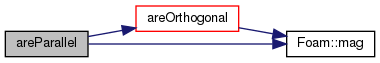Here is the caller graph for this function: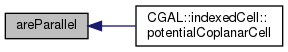## ◆ areOrthogonal()

 bool Foam::vectorTools::areOrthogonal ( const Vector< T > & a, const Vector< T > & b, const T & tolerance = `small` )

Test if a and b are orthogonal: a.b = 0.

Uses the dot product, so the tolerance is proportional to the cosine of the angle between a and b in radians

Definition at line 71 of file vectorTools.H.

References areAcute(), and Foam::mag().

Referenced by areParallel().

Here is the call graph for this function: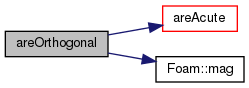Here is the caller graph for this function: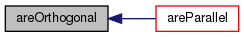## ◆ areAcute()

 bool Foam::vectorTools::areAcute ( const Vector< T > & a, const Vector< T > & b )

Test if angle between a and b is acute: a.b > 0.

Definition at line 83 of file vectorTools.H.

References areObtuse().

Referenced by areOrthogonal().

Here is the call graph for this function: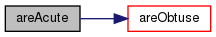Here is the caller graph for this function: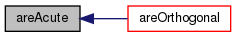## ◆ areObtuse()

 bool Foam::vectorTools::areObtuse ( const Vector< T > & a, const Vector< T > & b )

Test if angle between a and b is obtuse: a.b < 0.

Definition at line 94 of file vectorTools.H.

References cosPhi(), and Foam::T().

Referenced by areAcute().

Here is the call graph for this function: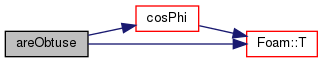Here is the caller graph for this function: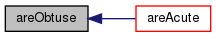## ◆ cosPhi()

 T Foam::vectorTools::cosPhi ( const Vector< T > & a, const Vector< T > & b, const T & tolerance = `small` )

Calculate angle between a and b in radians.

Definition at line 105 of file vectorTools.H.

Here is the call graph for this function: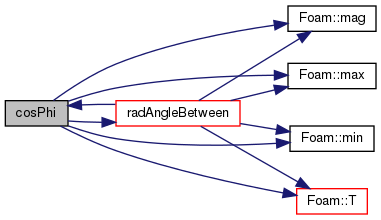Here is the caller graph for this function: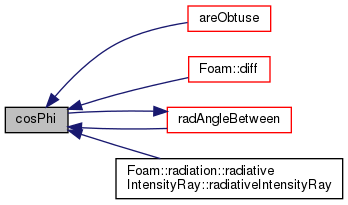## ◆ radAngleBetween()

 T Foam::vectorTools::radAngleBetween ( const Vector< T > & a, const Vector< T > & b, const T & tolerance = `small` )

Calculate angle between a and b in radians.

Definition at line 120 of file vectorTools.H.

Referenced by cosPhi(), and degAngleBetween().

Here is the call graph for this function: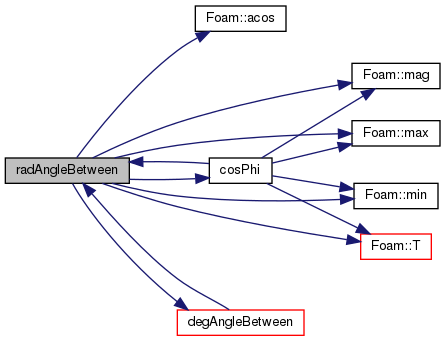Here is the caller graph for this function: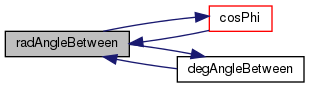## ◆ degAngleBetween()

 T Foam::vectorTools::degAngleBetween ( const Vector< T > & a, const Vector< T > & b, const T & tolerance = `small` )

Calculate angle between a and b in degrees.

Definition at line 135 of file vectorTools.H.

References radAngleBetween(), and Foam::radToDeg().

Referenced by radAngleBetween().

Here is the call graph for this function: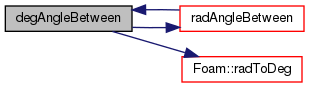Here is the caller graph for this function: